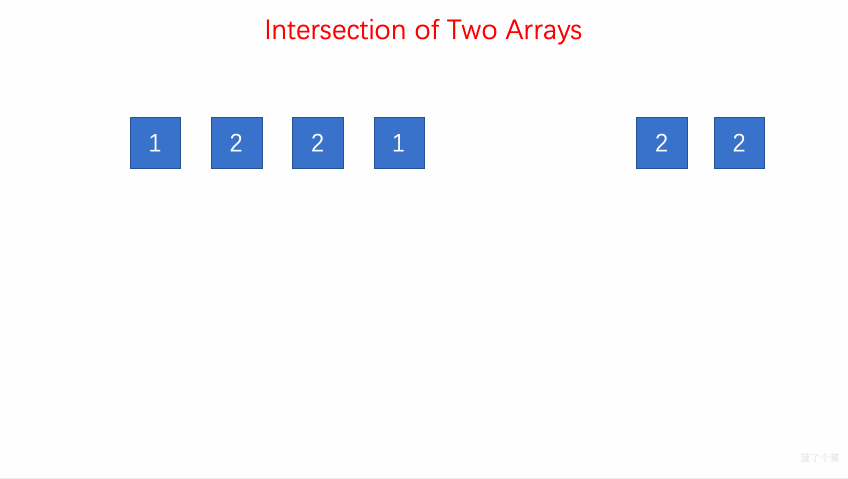# LeetCode 第 349 号问题：两个数组的交集

### 题目描述

``````输入: nums1 = [1,2,2,1], nums2 = [2,2]

``````

``````输入: nums1 = [4,9,5], nums2 = [9,4,9,8,4]

``````

• 输出结果中的每个元素一定是唯一的。
• 我们可以不考虑输出结果的顺序。

### 题目解析

• 遍历 num1，通过 set 容器 record 存储 num1 的元素
• 遍历 num2 ，在 record 中查找是否有相同的元素，如果有，用 set 容器 resultSet 进行存储
• 将 resultSet 转换为 vector 类型

### 动画描述### 代码实现

``````class Solution {
public:
vector<int> intersection(vector<int>& nums1, vector<int>& nums2) {
set<int> record;
for(int i = 0; i < nums1.size(); i ++){
record.insert(nums1[i]);
}

set<int> resultSet;
for(int i = 0; i < nums2.size();i++){
if(record.find(nums2[i]) != record.end()){
resultSet.insert(nums2[i]);
}
}

vector<int> resultVector;
for(set<int>::iterator iter=resultSet.begin(); iter != resultSet.end();iter++){
resultVector.push_back(*iter);
}
return resultVector;
}
};
``````